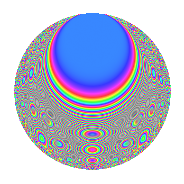# Properties

 Label 432.2.vLevel 432 Weight 2 Character orbit v Rep. character $$\chi_{432}(35,\cdot)$$ Character field $$\Q(\zeta_{12})$$ Dimension 88 Newform subspaces 1 Sturm bound 144 Trace bound 0

# Related objects

## Defining parameters

 Level: $$N$$ = $$432 = 2^{4} \cdot 3^{3}$$ Weight: $$k$$ = $$2$$ Character orbit: $$[\chi]$$ = 432.v (of order $$12$$ and degree $$4$$) Character conductor: $$\operatorname{cond}(\chi)$$ = $$144$$ Character field: $$\Q(\zeta_{12})$$ Newform subspaces: $$1$$ Sturm bound: $$144$$ Trace bound: $$0$$

## Dimensions

The following table gives the dimensions of various subspaces of $$M_{2}(432, [\chi])$$.

Total New Old
Modular forms 312 104 208
Cusp forms 264 88 176
Eisenstein series 48 16 32

## Trace form

 $$88q + 6q^{2} - 2q^{4} + 6q^{5} - 4q^{7} + O(q^{10})$$ $$88q + 6q^{2} - 2q^{4} + 6q^{5} - 4q^{7} - 8q^{10} + 6q^{11} - 2q^{13} + 6q^{14} - 2q^{16} - 8q^{19} + 48q^{20} - 2q^{22} + 12q^{23} + 8q^{28} + 6q^{29} + 6q^{32} + 2q^{34} - 8q^{37} + 6q^{38} - 2q^{40} - 2q^{43} - 40q^{46} - 24q^{49} - 72q^{50} - 2q^{52} - 16q^{55} - 36q^{56} + 16q^{58} + 42q^{59} - 2q^{61} - 44q^{64} + 12q^{65} - 2q^{67} - 96q^{68} - 16q^{70} - 78q^{74} - 14q^{76} + 6q^{77} - 36q^{82} - 54q^{83} + 8q^{85} - 54q^{86} + 22q^{88} + 20q^{91} - 108q^{92} + 6q^{94} - 4q^{97} + O(q^{100})$$

## Decomposition of $$S_{2}^{\mathrm{new}}(432, [\chi])$$ into newform subspaces

Label Dim. $$A$$ Field CM Traces $q$-expansion
$$a_2$$ $$a_3$$ $$a_5$$ $$a_7$$
432.2.v.a $$88$$ $$3.450$$ None $$6$$ $$0$$ $$6$$ $$-4$$

## Decomposition of $$S_{2}^{\mathrm{old}}(432, [\chi])$$ into lower level spaces

$$S_{2}^{\mathrm{old}}(432, [\chi]) \cong$$ $$S_{2}^{\mathrm{new}}(144, [\chi])$$$$^{\oplus 2}$$

## Hecke Characteristic Polynomials

There are no characteristic polynomials of Hecke operators in the database